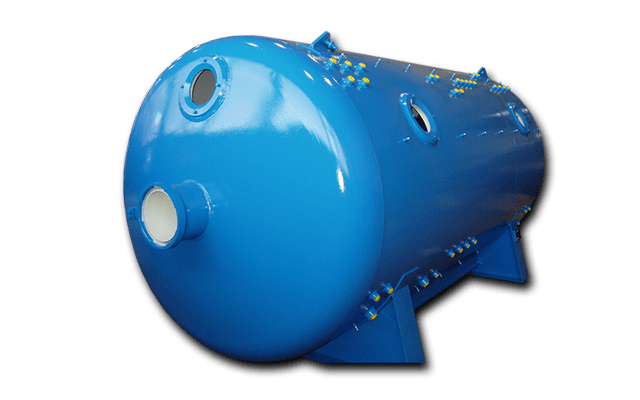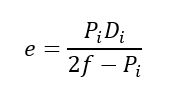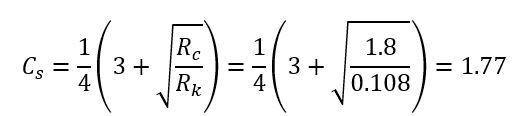# Example How to Design Thin-Walled Vessel under Internal Pressure

In this post, I want to share a simple example on how to design thin-walled vessel under internal pressure. Please check my previous post about the method on how to estimate minimum thickness of vessel component (shell, flat end closures, domed head closures).

## Example

Estimate the thickness required for the component parts of the vessel shown in the diagram.The vessel is to operate at a pressure of 16 bar (absolute) and design temperature of 300oC. The material of construction will be plain carbon steel. Welds will be fully radiographed. A corrosion allowance of 2 mm should be used.

## Solution

Design pressure, take as 10% above operating pressure = (16 – 1) × (1 + 10%) = 16.5 barg = 1.65 N/mm2

Design temperature = 300oC

From table below, typical design stress is 85 N/mm2.### Minimum thickness of cylindrical section

Minimum thickness of cylindrical section is calculated below.Where:

e         = minimum thickness (mm)

Pi        = internal pressure (N/mm2)

Di        = internal diameter of vessel (mm)

f         = design stress (N/mm2)

Calculation of minimum thickness of cylindrical section:

e = 1.65 × 1800 / (2 × 85 – 1.65) = 17.64 mm

Add corrosion allowance = 17.64 + 2 = 19.64 mm

1. Try a standard dished head (torisphere):

Crown radius Rc = Di = 1.8 m

Knuckle radius is 6% of Rc to prevent buckling = 0.108 m

A head of this size would be formed by pressing: no joints, so J = 1, then concentration factor (Cs) isMinimum thickness for dished head (torisphere) is calculated using equation below:Where:

Cs           = stress concentration factor for torispherical heads

For formed heads (heads without joints), the joint factor J is assumed to be 1.0.

Then, minimum thickness for dished head is:

e = (1.65 × 1800 × 1.77) / [2 × 85 × 1 + 1.65 × (1.77 – 0.2)] = 30.47 mm

2. For standard ellipsoidal head, ratio major:minor axes = 2:1

The minimal thickness necessary for this ratio can be determined using the equation below:Then, minimum thickness is:

e = (1.65 × 1800) / (2 × 1 × 85 – 0.2 × 1.65) = 17.50 mm

So, an ellipsoidal head would be probably the most economical.

For bolted cover with a full face gasket, take design constant Cp = 0.4

Take nominal plate diameter, De = 2 m

The minimum thickness of flat head is calculated using equation below:

Therefore, minimum thickness is:

e = (0.4 × 2000 × (1.65/85)0.5 = 111 mm

This shows inefficiency of flat cover. The required thickness will be large.